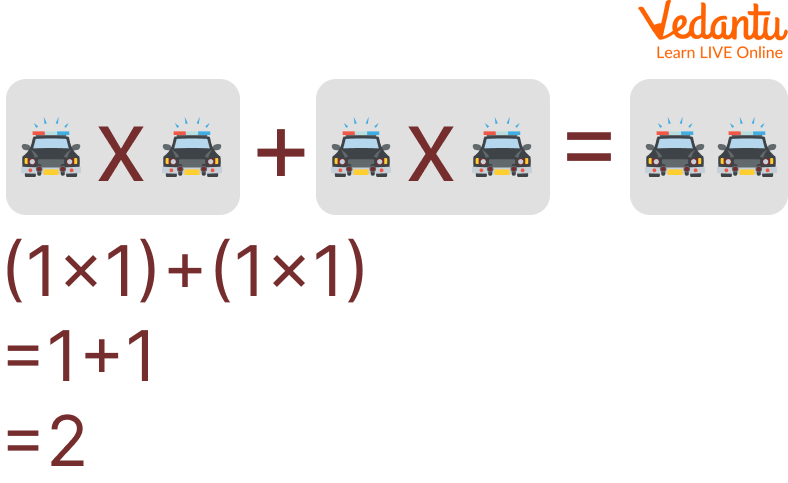Courses
Courses for Kids
Free study material
Offline Centres
More

# Product of SumLast updated date: 01st Dec 2023
Total views: 115.8k
Views today: 3.15k## Introduction to Product of Sum

Multiplication of two or more integers and the result we get is known as the Product of the Sum. It is a crucial component of mathematics and has many uses. The product of a Sum is a function that computes the product of two or more numbers. The function has been used in many mathematical problems like calculating the number of combinations for a given number, finding the probability distribution, and generating random numbers.

This article will explore using the Product of Sum in different scenarios and its applications. We will also explore how it can be used to generate more efficient and accurate results.Product of Sum

## What is the Sum of the Product?

The Product of Sum involves adding up the first number and then multiplying it by the sum of all other numbers and adding up all products. A sum of terms can be represented as each term being an integer greater than or equal to 0 and less than or equal to the number. For example, if we have three numbers: 5, 8, and 13, then we would calculate their product as follows:

$5\times 8 \times 13 = (5+8)\times(5 \times 8)$

$5 \times 8 \times 13=13\times(5 \times 8)=520$

This method is commonly used in mathematics for calculating products with large numbers, such as quadratic equations or polynomials. We'll utilize the Product of the Sum function to determine how many possible choices there are for a given integer. The product of Sum is a mathematical expression representing the total sum of all the terms in a series. It is denoted by the symbol " $\Sigma "$.

## Product of Sum-Related Formula

The following formula for the Product of Sum has been discussed:

$(X+Y)(X-Y)=X(X-Y)+Y(X-Y)$

$(X+Y)(X-Y)=X \times X-X \times Y+Y \times X-Y \times Y$

$(X+Y)(X-Y)=X^2-X Y+X Y-Y^2$

$(X+Y)(X-Y)=X^2-Y^2$

$\text { (First term - Second term) (First term }+\text { Second term) }=\text { First term }^2 \text { - Second term }{ }^2$Product of Sum Formula

## Product of Sum Example

The following examples of the Product of Sum have been explored in this article:

Q1. Find the product (3a + 5b) (3a – 5b) by using the identity.

Ans: We know that $(X+Y)(X-Y)=X^2-Y^2$

Where $X=3 a, Y=5 b$

Then, $(3 a+5 b)(3 a-5 b)=(3 a)^2-(5 b)^2$

$(3 a+5 b)(3 a-5 b)=3 \cdot 3 \cdot a \cdot a-5 \cdot 5 \cdot b \cdot b$

Therefore,

$(3 a+5 b)(3 a-5 b)=9 a^2-25 b^2$

Q2. Evaluate $8^2-7^2$ using the identity

Ans: We know that $(X+Y)(X-Y)=X^2-Y^2$

Where $X=8, Y=7$

Then,

$X^2-Y^2=(8+7)(8-7)$

$X^2-Y^2=(15)(1)$

Therefore,

$X^2-Y^2=15$

Q3. Simplify 35 × 25 by expressing it as the product of binomial sum and difference.

Ans: We know that $(X+Y)(X-Y)=X^2-Y^2$

Then, $35 \times 25=(30+5)(30-5)$

Where $X=30, Y=5$

$(X+Y)(X-Y)=30^2-5^2$

$(X+Y)(X-Y)=900-25$

$(X+Y)(X-Y)=875$

## Product of Sum Worksheet

Here the Worksheet related to the Product of the sum has been discussed, which is as follows:

Q1. Simplify $25 \times 15$ by expressing it as the product of binomial sum and difference.

Ans: 375

Q2. Using the identity, find the product $(6 a+2 b)(6 a-2 b)$.

Ans: $36 a^2-4 b^2$

Q3. Simplify $39 \times 21$ by expressing it as the product of binomial sum and difference.

Ans: 819

Q4. Evaluate $15^2-10^2$ using the identity

Ans: 125

Q5. Using the identity, find the product $(7 a+3 b)(7 a-3 b)$.

Ans: $49 a^2-9 b^2$

## Summary

There are many different uses of the Product of Sum. In this article, we have discussed the Product of the Sum formula in Math. We also gave some examples to explain the concept and its use cases. The formula for finding the product of the sum is $(X+Y)(X-Y)=X^2-Y^2$. In conclusion, we can say that the Product of Sum is a useful formula in Math that students can use to solve math problems. Teachers can also use it as a tool for teaching students how to solve math problems with different types of formulas.

## FAQs on Product of Sum

1. What are the properties of the product of the sum?

A product of a sum is the result obtained by multiplying two or more terms. For example, the product of the sum of three and four is 12.

The properties of the product of the sum are:

1. The product of the sum is always a positive number

2. The product of the sum can be expressed as a fraction

3. The product of the sum can be expressed as a decimal

4. The product of the sum cannot be found in the individual terms

5. No negative numbers exist in the real world that can be expressed as a product

2. What are some applications of the product of sum?

The product of sum is a formula that calculates the product of two or more numbers. The product of sum can also be used in other areas such as business, engineering, and science. It can be used to find the total number of parts and pieces needed for a project or to calculate how much it would cost to build an item with different parts.

3. How do you find the product of two numbers?

In math, the product of two numbers is found by multiplying the first number by the second. For example, $10 \times 10$ = 100.